Examples

Chapter 16 Class 11 Probability
Serial order wise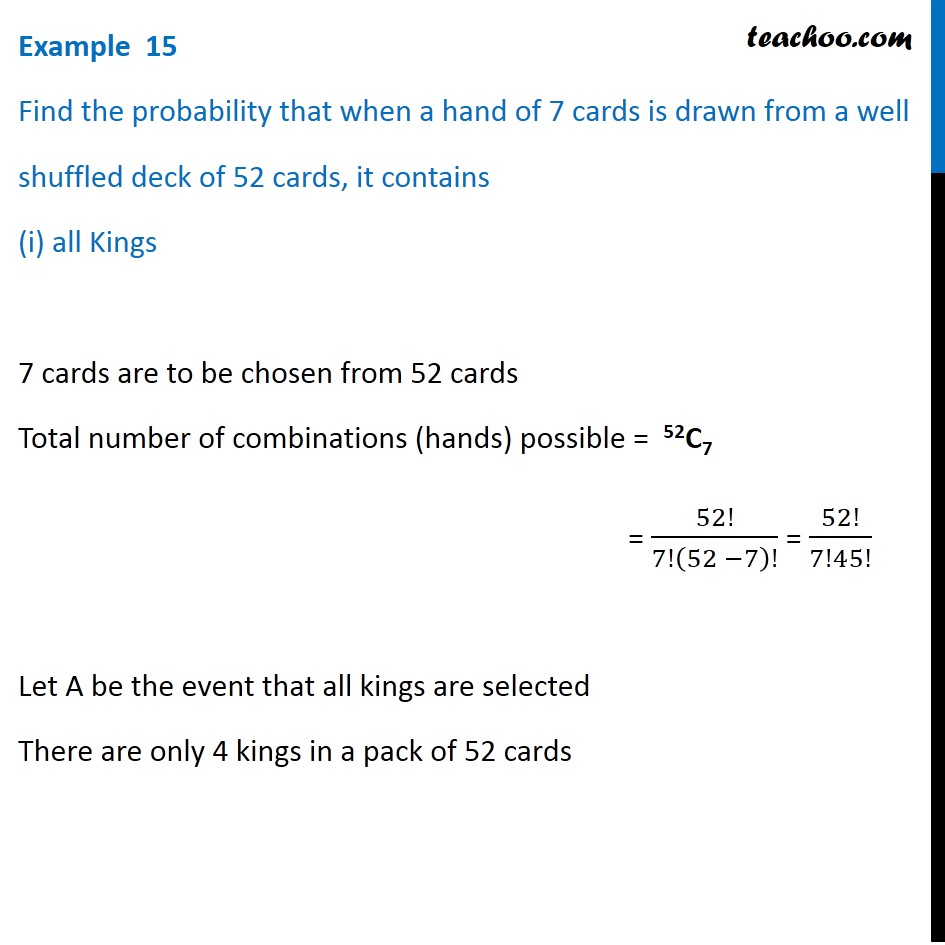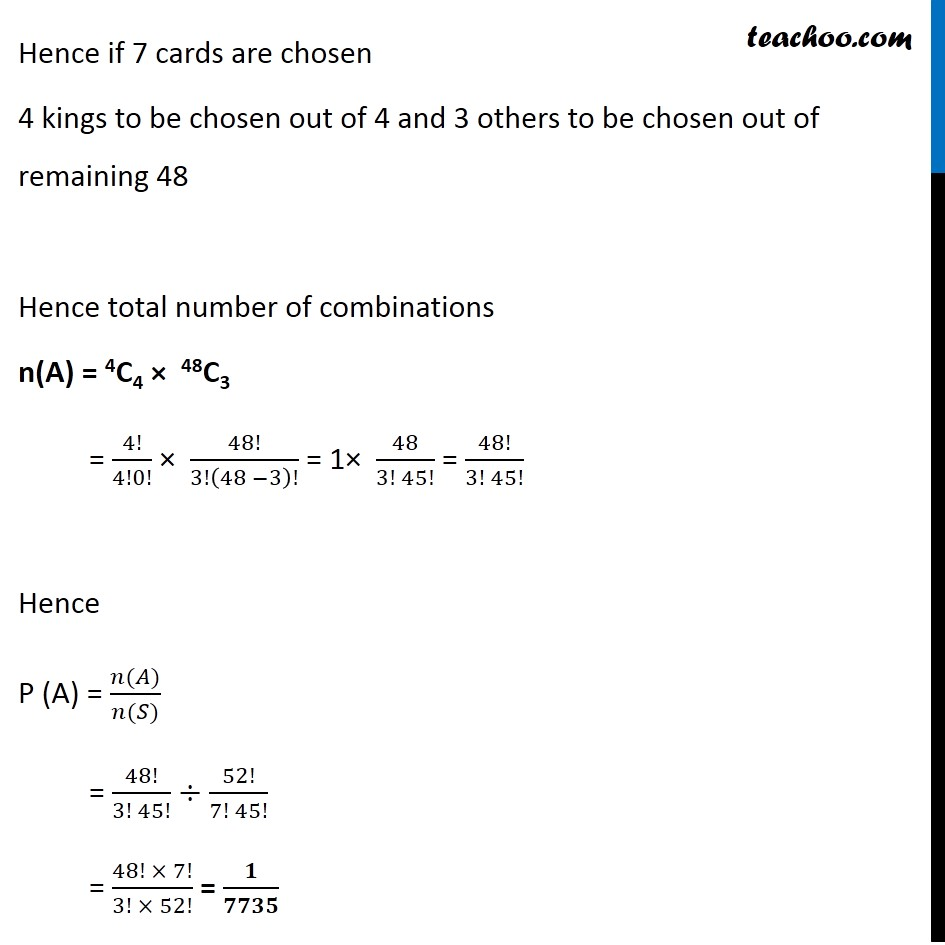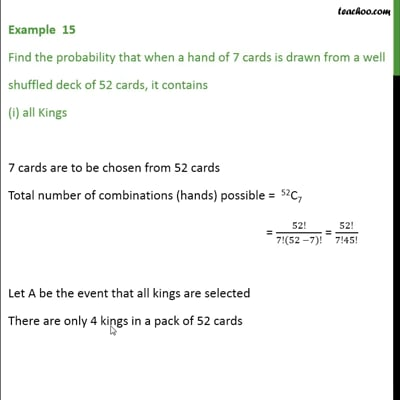This video is only available for Teachoo black users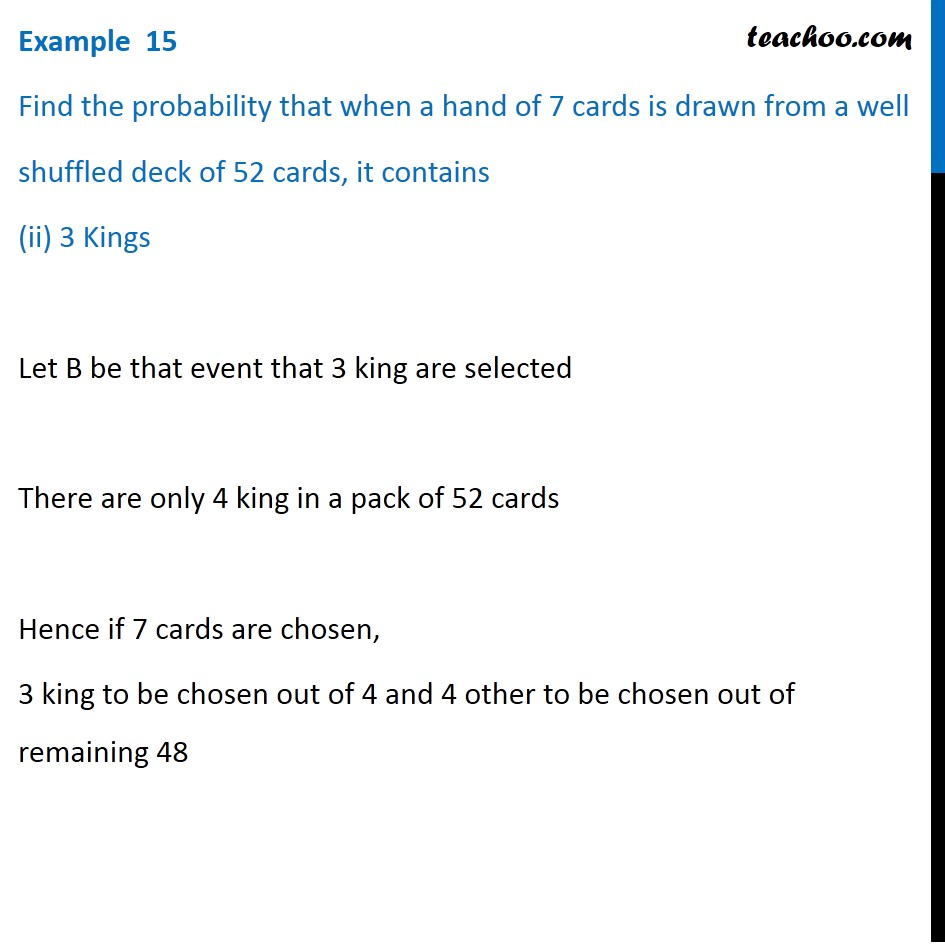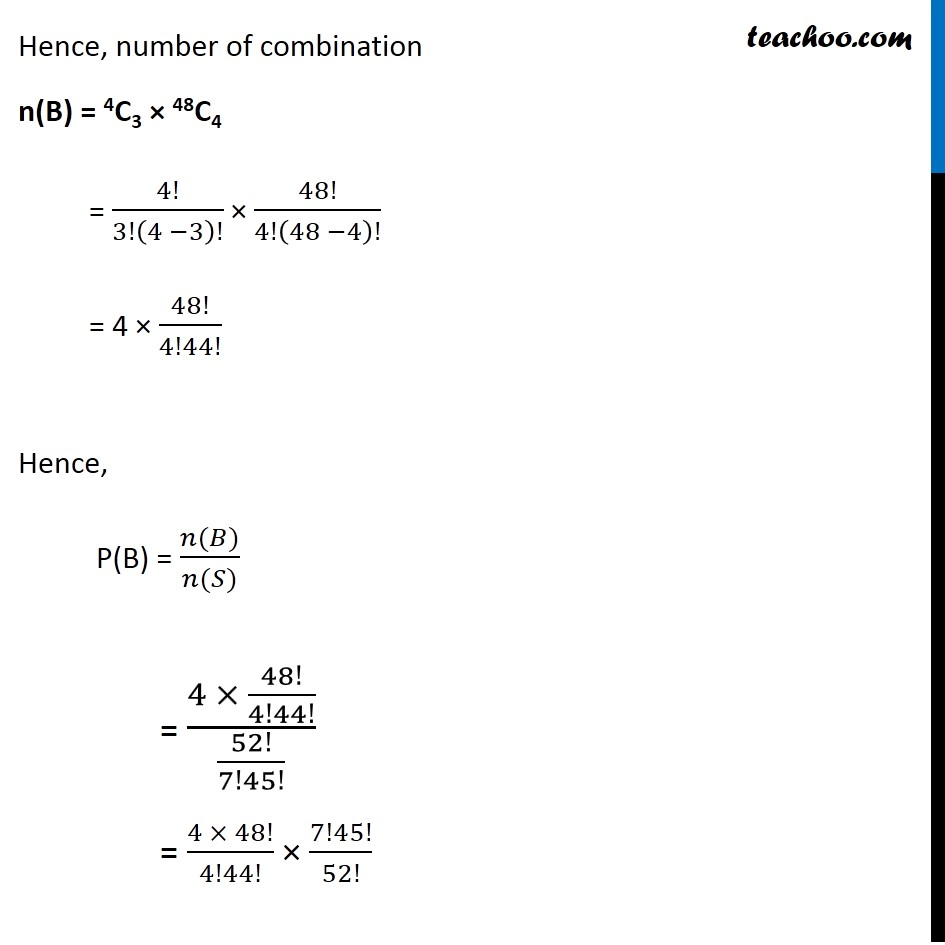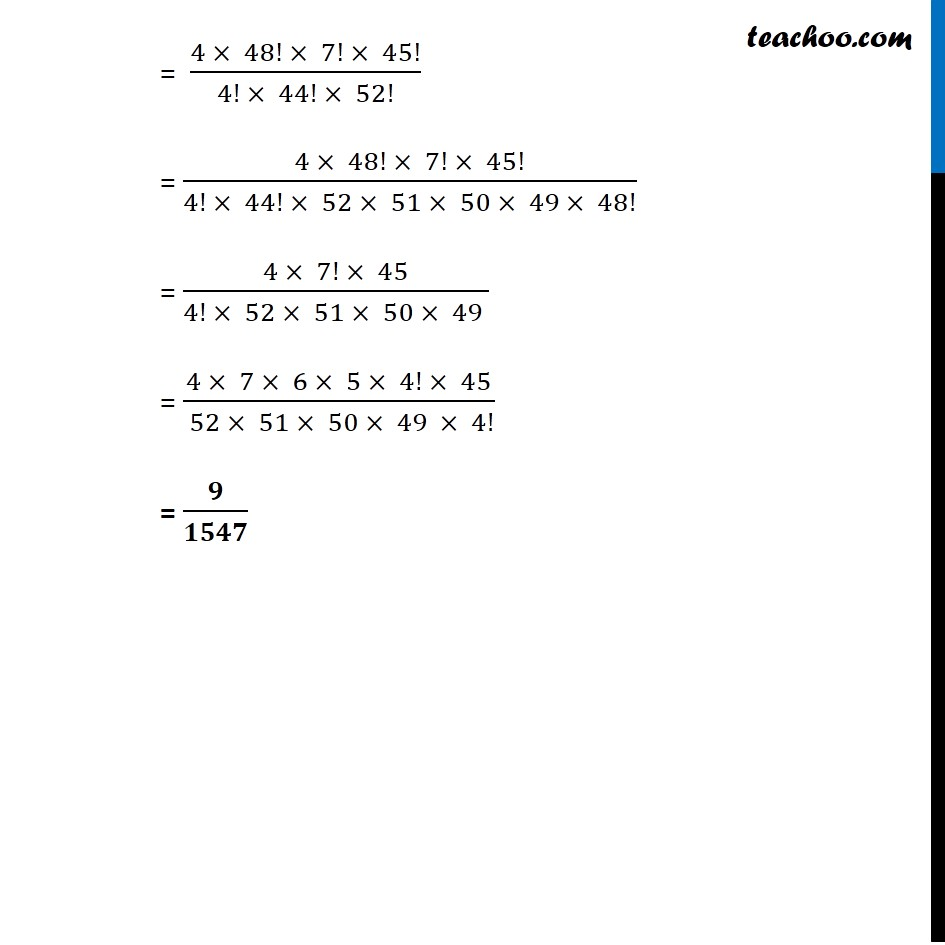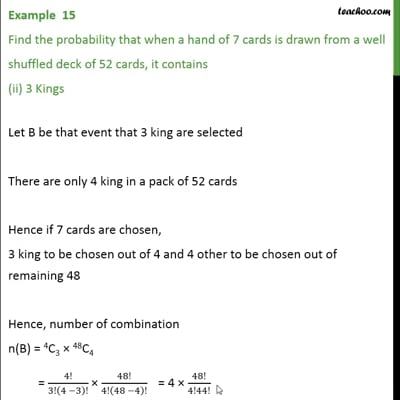This video is only available for Teachoo black users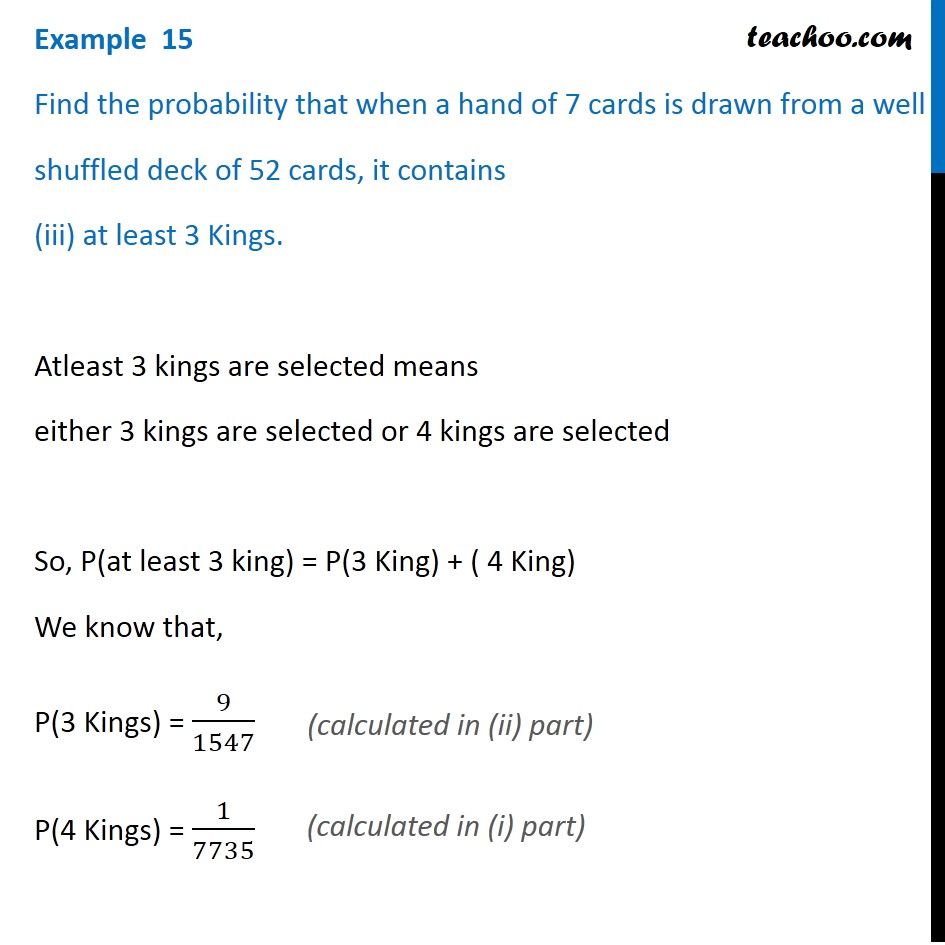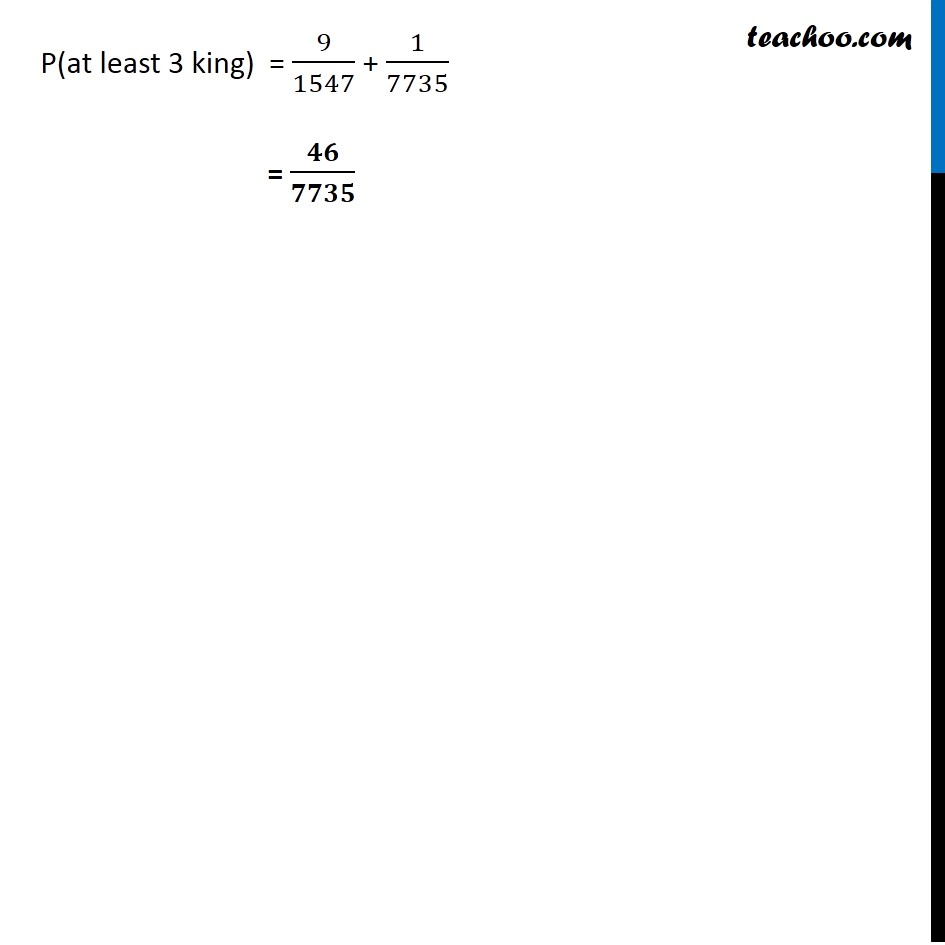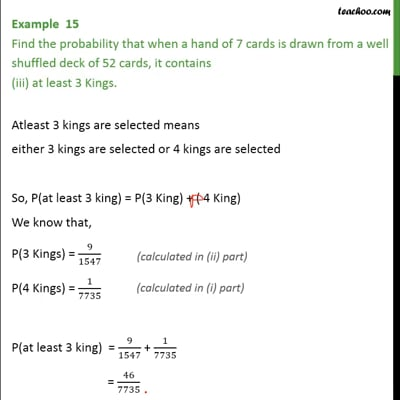This video is only available for Teachoo black users

Solve all your doubts with Teachoo Black (new monthly pack available now!)

### Transcript

Example 15 Find the probability that when a hand of 7 cards is drawn from a well shuffled deck of 52 cards, it contains (i) all Kings 7 cards are to be chosen from 52 cards Total number of combinations (hands) possible = 52C7 = 52!/7!(52 −7)! = 52!/7!45! Let A be the event that all kings are selected There are only 4 kings in a pack of 52 cards Hence if 7 cards are chosen 4 kings to be chosen out of 4 and 3 others to be chosen out of remaining 48 Hence total number of combinations n(A) = 4C4 × 48C3 = 4!/4!0! × 48!/3!(48 −3)! = 1× 48/(3! 45!) = 48!/(3! 45!) Hence P (A) = (𝑛(𝐴))/(𝑛(𝑆)) = 48!/(3! 45!) ÷ 52!/(7! 45!) = (48! × 7!)/(3! × 52!) = 𝟏/𝟕𝟕𝟑𝟓 Example 15 Find the probability that when a hand of 7 cards is drawn from a well shuffled deck of 52 cards, it contains (ii) 3 Kings Let B be that event that 3 king are selected There are only 4 king in a pack of 52 cards Hence if 7 cards are chosen, 3 king to be chosen out of 4 and 4 other to be chosen out of remaining 48 Hence, number of combination n(B) = 4C3 × 48C4 = 4!/3!(4 −3)! × 48!/4!(48 −4)! = 4 × 48!/4!44! Hence, P(B) = (𝑛(𝐵))/(𝑛(𝑆)) = (4 × 48!/4!44!)/(52!/7!45!) = (4 × 48!)/4!44! × 7!45!/52! = (4 × 48! × 7! × 45!)/(4! × 44! × 52!) = (4 × 48! × 7! × 45!)/(4! × 44! × 52 × 51 × 50 × 49 × 48!) = (4 × 7! × 45)/(4! × 52 × 51 × 50 × 49 ) = (4 × 7 × 6 × 5 × 4! × 45)/( 52 × 51 × 50 × 49 × 4!) = 𝟗/𝟏𝟓𝟒𝟕 Example 15 Find the probability that when a hand of 7 cards is drawn from a well shuffled deck of 52 cards, it contains (iii) at least 3 Kings. Atleast 3 kings are selected means either 3 kings are selected or 4 kings are selected So, P(at least 3 king) = P(3 King) + ( 4 King) We know that, P(3 Kings) = 9/1547 P(4 Kings) = 1/7735 (calculated in (ii) part) (calculated in (i) part) P(at least 3 king) = 9/1547 + 1/7735 = 𝟒𝟔/𝟕𝟕𝟑𝟓